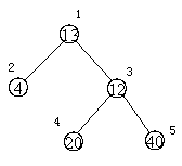# 医院设置

#### 题目

1处，则距离和=4+12+220+240=136
3处，则距离和=4*2+13+20+40=81input
5
13 2 3
4 0 0
12 4 5
20 0 0
40 0 0

output
81

SPFA

#### 代码

#include<iostream>
#include<cstring>
#include<cstdio>
using namespace std;
struct c{
int x,next;
}a;
void demo(int i){
memset(p,0,sizeof(p));
memset(d,0x7f,sizeof(d));
memset(f,0,sizeof(f));
p[i]=1;
f=i;
d[i]=0;
int h=0,t=1;
do{
h++;
if (d[f[h]]+1<d[a[j].x])  //松弛操作
{
d[a[j].x]=d[f[h]]+1;
if (p[a[j].x]==0)  //未进入队列
{
t++;
f[t]=a[j].x;
p[a[j].x]=1;
}
}
p[f[h]]=0;
}while (h<t);
}
int main()
{
scanf("%d",&n);
for (int i=1;i<=n;i++)
{
scanf("%d%d%d",&b[i],&x,&y);
if (x!=0)
{
t++;
a[t].x=x;
t++;
a[t].x=i;
}
if (y!=0)
{
t++;
a[t].x=y;
t++;
a[t].x=i;
}
}  //构建邻接表，无向图，若x,y为0不用存
for (int i=1;i<=n;i++)
{
int ans=0;
demo(i);  //枚举每个点做起点
for (int j=1;j<=n;j++)
ans+=b[j]*d[j];  //求距离和
mi=min(ans,mi);
}
cout<<mi<<endl;
return 0;
}


©️2019 CSDN 皮肤主题: 游动-白 设计师: 上身试试